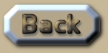Science of the gaps

 Science of the Gaps A common knee-jerk reaction to the planetary-geometry that evolves in these algorithms made by the typical anonymous internet troll is that: ‘Other aspects of Relativity compensate’ for the drift that these algorithms compute. It is simply assumed that Relativity is correct, and that the ‘gaps’ demonstrated in this assessment are filled in by ‘Science’. It is deeply ironic how most of the scientific community mimics the naïve part of the Religious community in its assumptions. .
 If gravity moves at the velocity of light then Jupiter would move away from the Sun at hundreds of kilometres per orbit. The Alpha Centauri binary would move away from each other at over a million kilometres per orbit – if gravity moved at the velocity of light. There simply is no other part of Relativity that can compensate for this massive fluctuation. The computational cause of these drifts away from the Newtonian orbit is simply a geometric consequence of gravity (hypothetically) moving at the velocity of light. Under the same concept, the Moon would move away from the Earth at more than 5km per year. All the other facets of Relativity are measured as being in the vicinity of 1mm per lunar orbit – or less. The difference here is a factor of nearly half a million times. The outwards movement of the orbit caused by gravity having velocity (General Relativity) is actually half a million times greater than any other aspect of Relativity in the context of the lunar orbit. The gravity of other planets (and the Sun), being beyond the orbit of the Moon can only add to the outwards drift of the Moon away from the Earth. So those who try and apply assumptions of n-body gravity to the orbit of the Moon without constructing an evolutionary algorithm are similarly guilty of ‘Science of the Gaps’. Simply being able to place numbers in a formula is certainly the ‘illusion of explanation’. Now what about the pervasive claims that GPS uses Relativity? Simply: It does not. That is just a popular internet rumour endlessly regurgitated by the scholastic mob. It was certainly demonstrated to be without any logical foundation in Chapter XXVIII which also contains several references to companies that use GPS systems, all of which refute the use of Relativity in their operations. That chapter also demonstrates numerous other mathematical reasons as to why GPS could not use Relativity. .This is an extract summary of Chapter XXX of the book: Flight Light and Spin